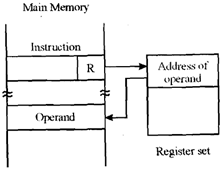## Explain register indirect addressing, Computer Engineering

Assignment Help:

In this addressing technique the operand is data in memory pointed to by a register. Or we can say in other words that the operand field specifies a register whichcomprises the address of operand which is stored in memory. This is almost similar to indirect addressing scheme except it is much faster than indirect addressing which requires two memory accesses.#### Write a short note on structure chart, Write a short note on structure char...

Write a short note on structure chart.    Structure Chart is an significant program design method and it shows all components of code in a hierarchical format

#### The need of parallel computation, The Need Of Parallel Computation With...

The Need Of Parallel Computation With the advancement of computer science (CS), computational speed of the processors has also improved many a time. Moreover, there is certain

#### We declare a table control in the abap/4 program, How can we declare a tabl...

How can we declare a table control in the ABAP/4 program? Using the syntax controls type tableview using screen .

#### Canonical genetic algorithm - mating, Canonical genetic algorithm - Mating:...

Canonical genetic algorithm - Mating: In such a scenario this continues until the number of offspring that is produced is the required number. Further this required number is

#### Solve out linear equations, Assume that you have been asked to solve proble...

Assume that you have been asked to solve problem with exact area constraints, the area error being no more than 1% for each department. What are the linear equations you would nee

#### Flowchart, How to calculate the flowchart

How to calculate the flowchart

#### Explain program level of parallel processing, Program Level This is nor...

Program Level This is normally the liability of OS (operating system) that runs processes simultaneously. Different programs are generally independent of each other. So paralle

#### What is dhcp and how it works, Full form of is DHCP: Dynamic Host Control P...

Full form of is DHCP: Dynamic Host Control Protocol. It is mainly used to bound MAC Addresses of system. Especially for Wireless System.

#### Difference between relocatable and self relocatable programs, Difference be...

Difference between relocatable and self relocatable programs. A relocatable program is one which can be processed to relocate it to a selected area of memory. For illustratio

#### Why a task cannot return a value, Why a task can n ot return a value? ...

Why a task can n ot return a value? If tasks can return values then Let's take a look at the below example. A=f1(B)+f2(C); and f1 and f2 had delays of say 5 and 10? Whe